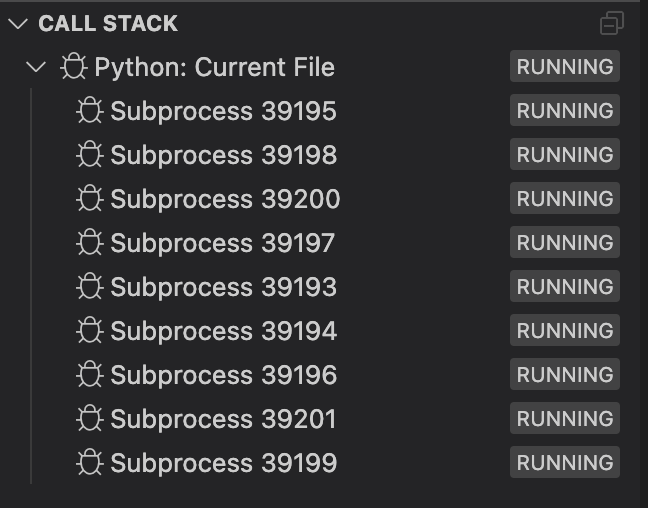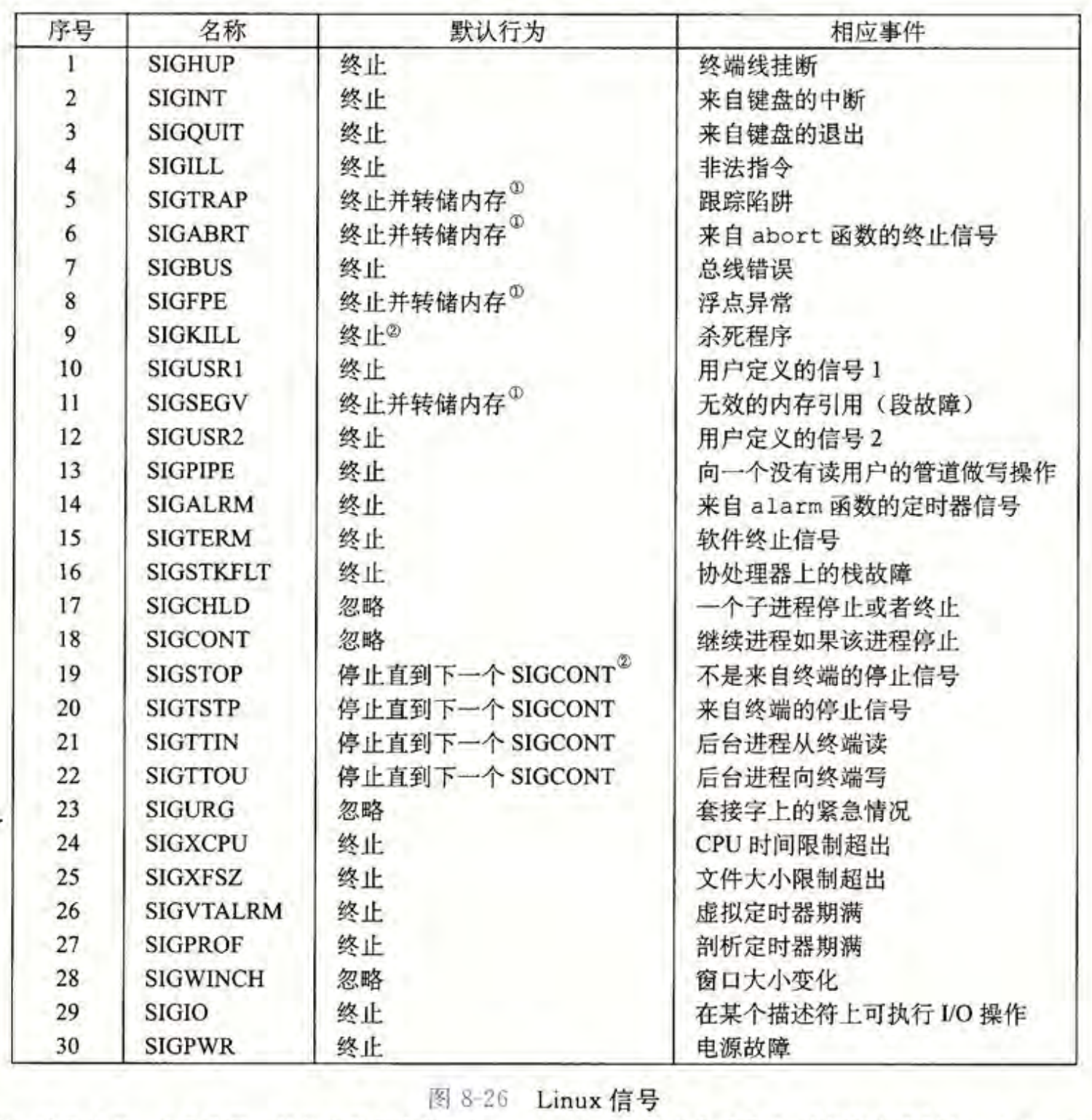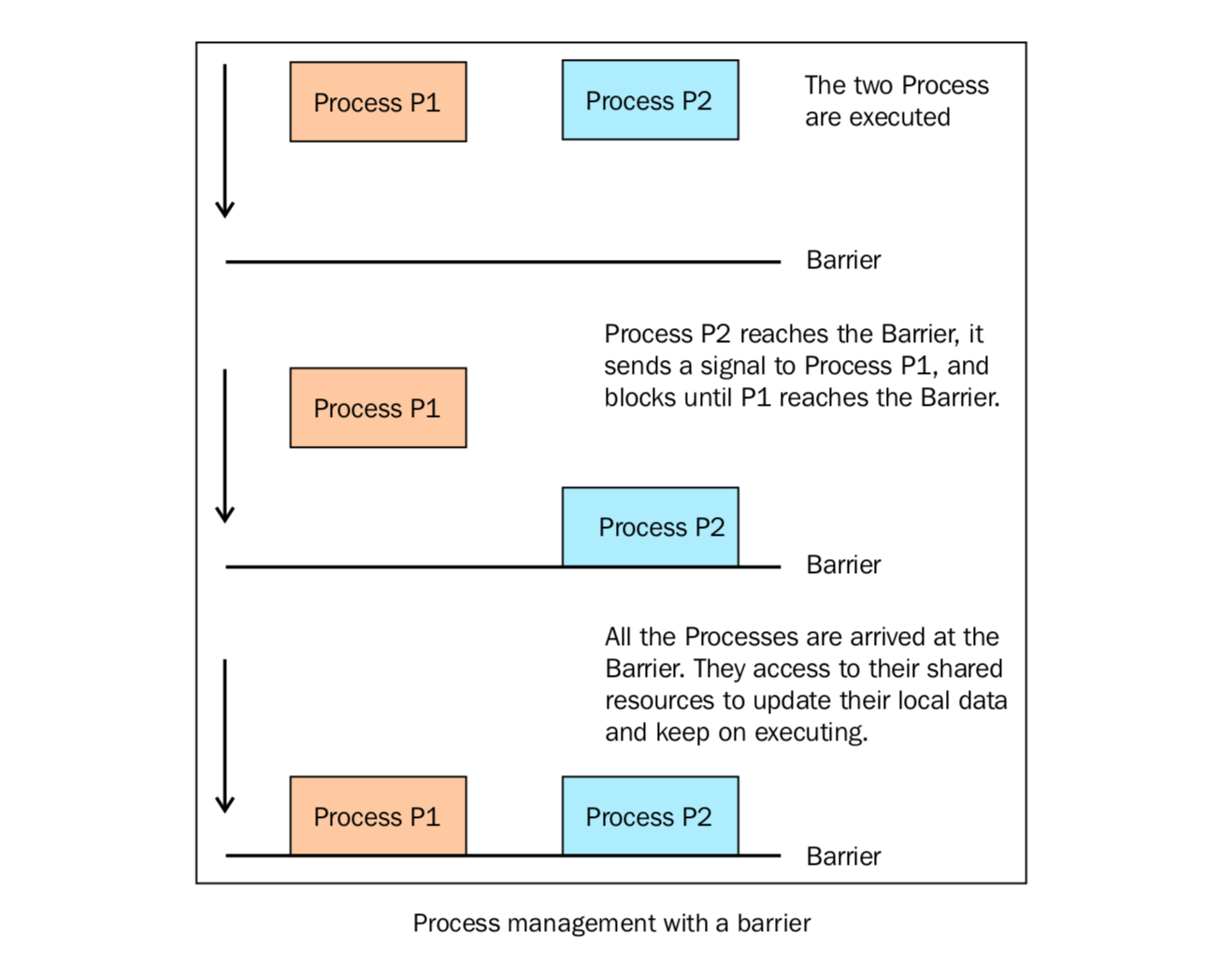# Python：多进程并行编程与进程池

Python的并行编程可以采用multiprocessingmpi4py模块来完成。

multiprocessing是Python标准库中的模块，实现了共享内存机制，也就是说，可以让运行在不同处理器核心的进程能读取共享内存。在基于共享内存通信的多进程编程中，常常通过加锁或类似机制来实现互斥。

mpi4py库实现了消息传递的编程范式（设计模式）。简单来说，就是进程之间不靠任何共享信息来进行通信（也叫做shared nothing），所有的交流都通过传递信息代替。在信息传递的代码中，进程通过send()receive()进行交流（与共享内存通信形成对比）。

# 1 Unix进程模型回顾

Python的multiprocessing进程模块在Unix上是基于fork()编程接口的(MacOS上默认是spawn())，故在介绍Python的并行编程模块之前，首先让我们回顾一下Unix的进程模型。

• 一个独立的逻辑控制流，它提供一个假象，好像我们的程序独占地使用处理器。
• 一个私有的地址空间，它提供一个假象，好像我们的程序独占地使用内存系统。

Unix系统上常通过以下方式创建子进程：

# include <stdlib.h>
# include <unistd.h>
# include <stdio.h>

int main(){
pid_t pid;
int x = 1;
pid = fork();
if (pid == 0){ //Child
printf("child: x=%d\n", ++x);
exit(0);
}
//Parent
printf("parent: x=%d\n", --x);
exit(0);
}


parent: x=0
child: x=2


fork()函数是一点需要注意的事它只被调用一次，却会返回两次：一次是在调用进程（父进程）中，一次是在新创建的子进程中。在父进程中，fork()返回子进程的PID；在子进程中，fork() 返回0。因为子进程的PID总是为非零，返回值就提供一个明确的方法来分辨程序是在父进程还是在子进程中执行。

# 2 用multiprocessing模块创建进程

1. 创建进程对象
2. 调用start()方法，开启进程的活动
3. 调用join()方法，在进程结束之前一直等待。

import multiprocessing
from multiprocessing import shared_memory
import os
import time

def foo(i, data):
data[i] = i
print ('called function in process: %d, data[%d] = %d' % (os.getpid(), i, data[i]))
time.sleep(10)
return

if __name__ == '__main__':

n_processes = 8
data = [None for i in range(n_processes)]

begin = time.time()
Process_jobs = []
for i in range(n_processes):
# 每个子进程都拿了一个data副本
p = multiprocessing.Process(target=foo, args=(i, data))
Process_jobs.append(p)
p.start()

for i in range(n_processes):
Process_jobs[i].join()
end = time.time()

print(data)
print("time: %d" % (end - begin))


called function in process: 39195, data = 1
called function in process: 39198, data = 4
called function in process: 39200, data = 6
called function in process: 39197, data = 3
called function in process: 39201, data = 7
called function in process: 39196, data = 2called function in process: 39194, data = 0

called function in process: 39199, data = 5
[None, None, None, None, None, None, None, None]
time: 11# 3 共享内存

import multiprocessing
from multiprocessing import shared_memory
import os
import time

def foo(i, data):
data[i] = i
print ('called function in process: %d, data[%d] = %d' % (os.getpid(), i, data[i]))
time.sleep(10)
return

if __name__ == '__main__':

n_processes = 8
data = [None for i in range(n_processes)]
# 需要置为共享内存
data = shared_memory.ShareableList([999 for i in range(n_processes)])

begin = time.time()
Process_jobs = []
for i in range(n_processes):
# 每个子进程都拿了一个data副本
p = multiprocessing.Process(target=foo, args=(i, data))
Process_jobs.append(p)
p.start()

for i in range(n_processes):
Process_jobs[i].join()
end = time.time()

print(data)
print("time: %d" % (end - begin))

data.shm.close()



called function in process: 43848, data = 6
called function in process: 43842, data = 0
called function in process: 43849, data = 7
called function in process: 43843, data = 1
called function in process: 43846, data = 4
called function in process: 43844, data = 2
called function in process: 43847, data = 5
called function in process: 43845, data = 3
ShareableList([0, 1, 2, 3, 4, 5, 6, 7], name='psm_c252aac3')
time: 11


# 4 终止/杀掉进程

# 杀死一个进程
import multiprocessing
import time

def foo():
print('Starting function')
time.sleep(0.1)
print('Finished function')

if __name__ == '__main__':
p = multiprocessing.Process(target=foo)
print('Process before execution:', p, p.is_alive())
p.start()
print('Process running:', p, p.is_alive())
p.terminate()
time.sleep(0.1) # 注意，传信号需要时间，故sleep一下
print('Process terminated:', p, p.is_alive())
p.join()
print('Process joined:', p, p.is_alive())
print('Process exit code:', p.exitcode)


Process before execution: <Process name='Process-1' parent=50932 initial> False
Process running: <Process name='Process-1' pid=50944 parent=50932 started> True
Process terminated: <Process name='Process-1' pid=50944 parent=50932 stopped exitcode=-SIGTERM> False
Process joined: <Process name='Process-1' pid=50944 parent=50932 stopped exitcode=-SIGTERM> False
Process exit code: -15


• == 0: 没有错误正常退出
• > 0: 进程有错误，并以此状态码退出
• < 0: 进程被-1 *信号杀死并以此作为 ExitCode 退出
在我们的例子中，输出的ExitCode-15 。负数表示子进程被数字为15的信号杀死。

Process before execution: <Process name='Process-1' parent=52481 initial> False
Process running: <Process name='Process-1' pid=52492 parent=52481 started> True
Process terminated: <Process name='Process-1' pid=52492 parent=52481 stopped exitcode=-SIGKILL> False
Process joined: <Process name='Process-1' pid=52492 parent=52481 stopped exitcode=-SIGKILL> False
Process exit code: -9


Unix系统上常见信号查询手册如下：# 5 进程间同步

Lock: 这个对象可以有两种状态：锁住的（locked）和没锁住的（unlocked）。一个Lock对象有两个方法， acquire()release() ，来控制共享数据的读写权限。
Event: 实现了进程间的简单通信，一个进程发事件的信号，另一个进程等待事件的信号。 Event对象有两个方法， set()clear()，来管理自己内部的变量。
Condition: 此对象用来同步部分工作流程，在并行的进程中，有两个基本的方法： wait()用来等待进程， notify_all()用来通知所有等待此条件的进程。
Semaphore: 用来共享资源，例如，支持固定数量的共享连接。
Rlock: 递归锁对象。其用途和方法同Threading模块一样。
Barrier: 将程序分成几个阶段，适用于有些进程必须在某些特定进程之后执行。处于障碍（Barrier）之后的代码不能同处于障碍之前的代码并行。

import multiprocessing
from multiprocessing import Barrier, Lock, Process
from time import time
from datetime import datetime

def test_with_barrier(synchronizer, serializer):
name = multiprocessing.current_process().name
synchronizer.wait()
now = time()
with serializer:
print("process %s ----> %s" % (name, datetime.fromtimestamp(now)))

def test_without_barrier():
name = multiprocessing.current_process().name
now = time()
print("process %s ----> %s" % (name, datetime.fromtimestamp(now)))

if __name__ == '__main__':
synchronizer = Barrier(2) # Barrier声明的参数代表要管理的进程总数：
serializer = Lock()
Process(name='p1 - test_with_barrier', target=test_with_barrier, args=(synchronizer,serializer)).start()
Process(name='p2 - test_with_barrier', target=test_with_barrier, args=(synchronizer,serializer)).start()
Process(name='p3 - test_without_barrier', target=test_without_barrier).start()
Process(name='p4 - test_without_barrier', target=test_without_barrier


process p3 - test_without_barrier ----> 2022-12-09 20:09:18.382580
process p1 - test_with_barrier ----> 2022-12-09 20:09:18.432348
process p2 - test_with_barrier ----> 2022-12-09 20:09:18.432586
process p4 - test_without_barrier ----> 2022-12-09 20:09:18.464562# 6 使用进程池

import time
import multiprocessing

def function_square(x):
return x * x

if __name__ ==  '__main__':
data = [i for i in range(1000000)]

# Parallel implementation
begin = time.time()
pool = multiprocessing.Pool(processes=8)
results1 = pool.map(function_square, data)
pool.close()
pool.join()
end = time.time()
print("Parallel time: %f" % (end - begin))

# Nonparallel code
begin = time.time()
results2 = map(function_square, data)
end = time.time()
print("Nonparallel time: %f" % (end-begin))

if list(results1) == list(results2):
print("Correct!")
print(results1[:10])


Parallel time: 1.638756
Nonparallel time: 0.000003
Correct!
[0, 1, 4, 9, 16, 25, 36, 49, 64, 81]


• 这种并行化处理技术只适用于可以将问题分解成各个独立部分的情况。
• 任务必须定义成普通的函数来提交。实例方法、闭包或者其他类型的可调用对象都是不支持并行处理的。
• 函数的参数和返回值必须课兼容于pickle编码。任务的执行是在单独的解释器中完成的，这中间需要用到进程间通信。因此，在不同的解释器间交换数据必须要进行序列化处理（这一点尤其重要，在实际项目中我发现Pytorch的sparse_coo_tensor(类型就无法进行序列化），在调用p.start()时会报错NotImplementedError: Cannot access storage of SparseTensorImpl

# 7 用多进程来规避GIL

# Performs a large calculation (CPU bound)
def some_work(args):
...
return result

# A thread that calls the above function
while True:
...
r = some_work(args)
...


import os

# Processing pool (see below for initiazation)
pool = None

#  Performs a large calculation (CPU bound)
def some_work(args):
...
return result

# A thread that calls the  above function
while True:
...
r = pool.apply(some_work, (args))
...

# Initiaze the pool
if __name__ == "__main__":
import multiprocessing
pool = multiprocessing.Pool()


# 参考

posted @ 2022-12-10 12:17  orion-orion  阅读(105)  评论(0编辑  收藏  举报